# The Only Guide You Need On: How To Count Unique Values In Excel

Jul 04, 2023Do you want to learn how to count values in Excel for easier data analysis and management?

Counting unique values in Excel doesn't have to be complicated. If you're ready to streamline your workflow and get more out of your spreadsheets, this blog post is for you.

We'll explain the steps necessary to count unique values in Excel and give some pro tips on making the task easier.

Read on as we cover the following:

• The Unique and Distinct Values in Excel

• Count Unique Values by Combining the SUM, IF, and COUNTIF Functions

• Count the Distinct Values in Excel

• Final Thoughts on How to Count Unique Values in Excel

• Frequently Asked Questions on How to Count Unique Values in Excel## The Unique and Distinct Values in Excel

When dealing with a lot of data, manually counting values can be nearly impossible. This is especially true when you're trying to keep track of unique or distinct values. However, Excel has many ways to count unique and distinct values.

You can classify the data into two categories: repeated and unrepeated. The unrepeated data type will divide into unique and distinct subcategories.

• Unique data in a dataset refers to values that appear only once.

• Distinct data includes duplicate values but only counts them once.

## Count Unique Values by Combining the IF, SUM, and COUNTIF Functions

Excel has functions such as SUM, IF, and COUNTIF that you can use together to count unique values.

To count unique values in Excel, enter the following formula: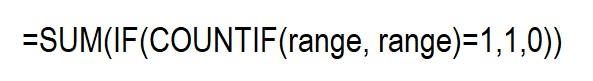For clarification, the range refers to the first and last cells you'll include. This formula stores count values in a new array and need to enter as an array formula.

Remember to press Ctrl+Shift+Enter after inputting the formula. The formula will automatically populate with curly braces at the end, so do not manually enter them.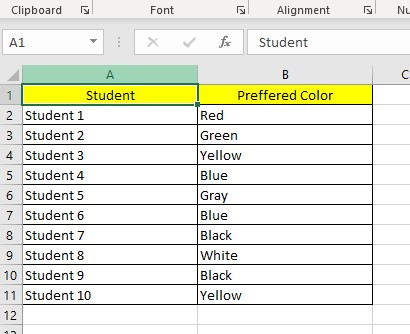1. Select a cell and type the SUM, IF, and COUNTIF formulas.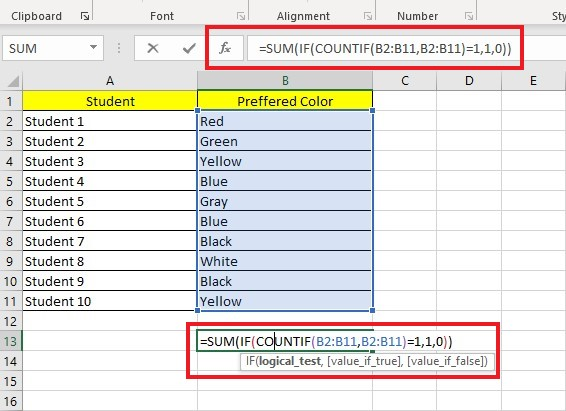1. Press the Enter key to get the unique values.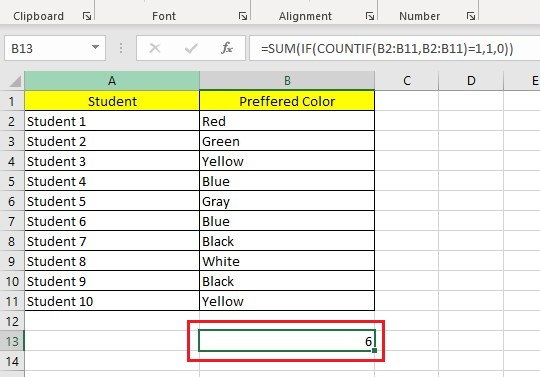The formula above will provide you with unique values. I will explain how to achieve this in three easy steps.

• The COUNTIF function calculates how many times each value in B2 to B11 appears and stores the counts in an array, which only includes unique values.

• Theretains only the values equal to 1 and changes all other values to 0.

• Finally, the SUM function adds the distinct value and outputs the result, which is 6.

You can use Excel's SUM, IF, and COUNTIF functions to find unique text or numeric values.

### Count the Unique Text Values.

You can modify the function to find unique text values in Excel tables and worksheets that contain both text and numbers.

1. Select a cell and type the Count Text Values formula.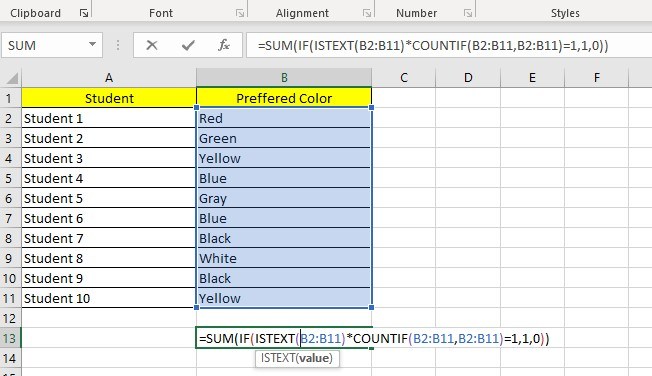1. Press the Enter key to get the results.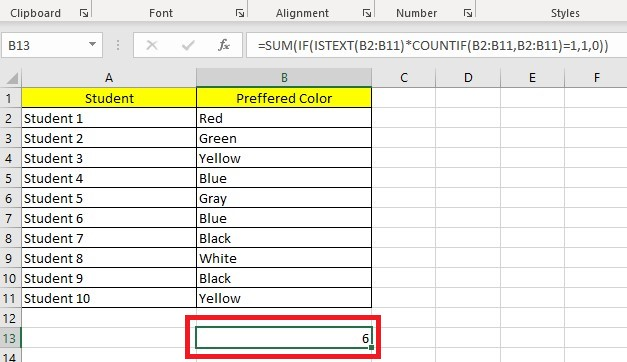We added the ISTEXT function to the general formula to identify unique text values. The ISTEXT function checks if the value is text. If it is, it adds 1 to an array. If the cell contains a non-text value, it adds a 0.

### Count the Unique Numeric Values

If you want to count unique numeric values included with texts, you can use the formula below.

To obtain the sum of values within a range, use the formula: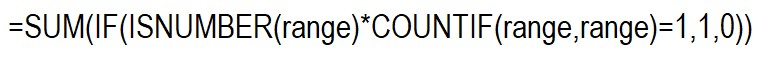Enter it into the cell where you want the result to show. In the formula, replace "range" with the starting and ending cells that contain the values.

Please note that in Excel, dates and times are also considered numbers.

You can use the methods below to count distinct values in Excel and count unique values, as we have previously demonstrated.

## Count the Distinct Values in Excel

Here are the different ways to count the distinct values in Excel.

### Count Distinct Values with the Filter Option

One way to obtain unique values for your data in Excel is by using the Filter option.

This method involves filtering out the distinct values into a separate row and then using the ROWS function to count the number of unique elements. This technique is straightforward and uncomplicated.

1. Select a column that has duplicate values.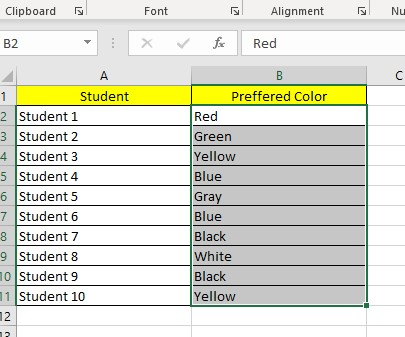1. Navigate to the Data tab, go to the Sort & Filter Group, and click Advanced.1. In the Advanced Filter dialog box, select an Action and List range.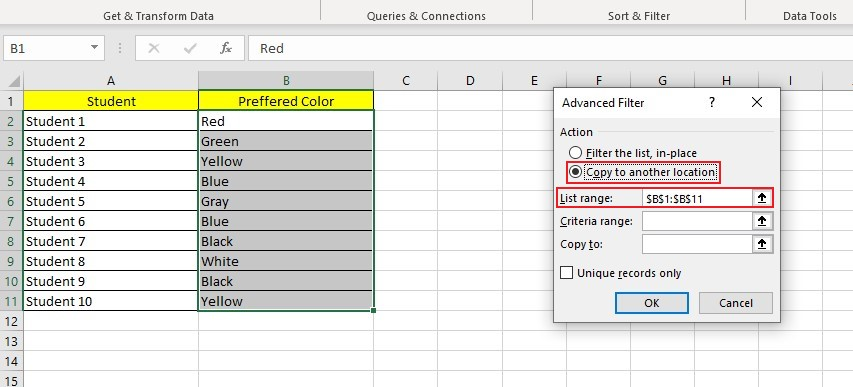1. Choose a cell where you want to put your unique values in the Copy to the text box and check Unique Records only.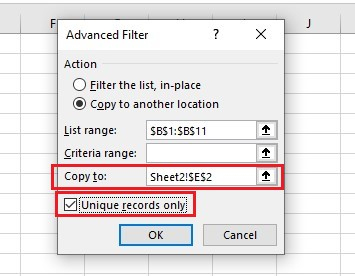The unique elements will duplicate onto a new column.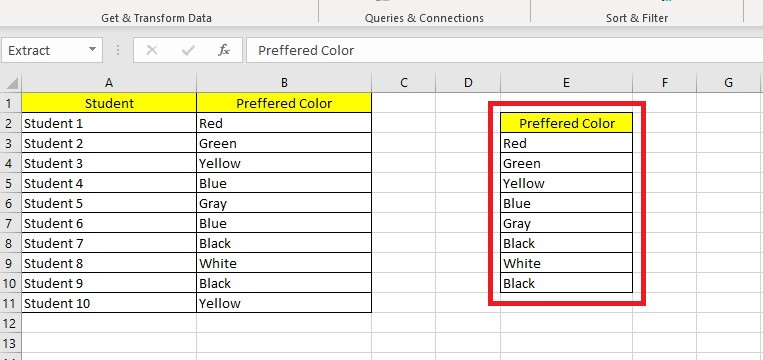To find the number of rows in a table, follow these steps:

1. Select the columns you want to total.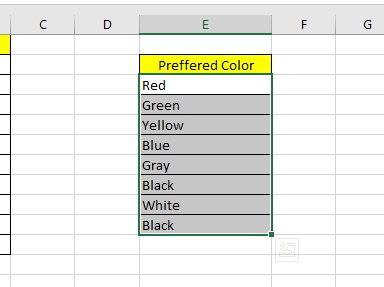1. Use the shortcut Ctrl + Q or click on the Quick Analysis button.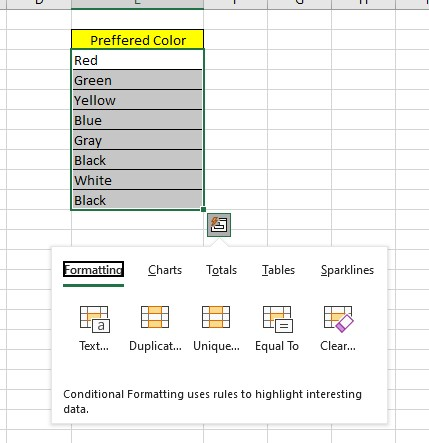1. Choose Totals and then click on Row Count.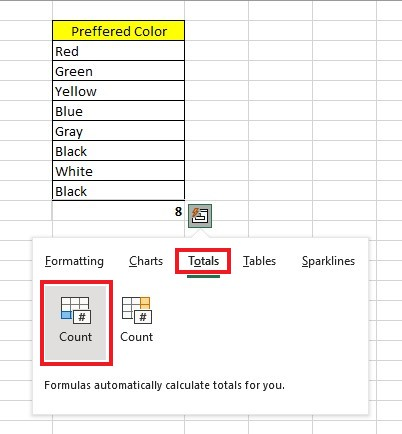The number of rows will appear below the unique elements.

To specify a range of cells, use the ROW function with the starting cell in "A" and the ending cell in "B."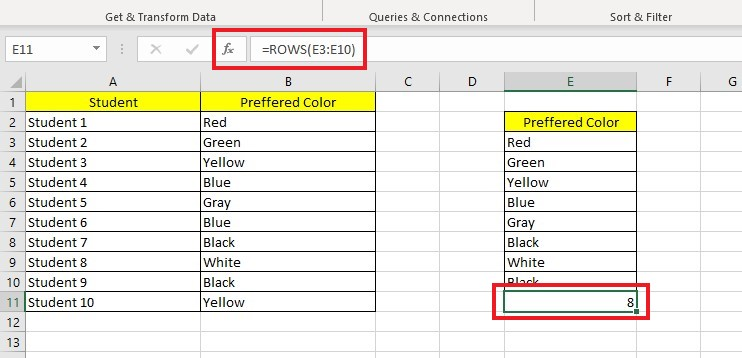Clicking the "Filter the list" option will replace the selected values in the same column without opening a new window.

### Count Distinct Values with SUM and COUNTIF Functions

To find distinct values in Excel, use the SUM and COUNTIF functions. In this scenario, we will use the inverse of the COUNTIF function to obtain distinct values.

To count distinct values with SUM and COUNTIF functions, follow the steps below:

1. Select a cell, then enter the SUM and COUNTIF formulas.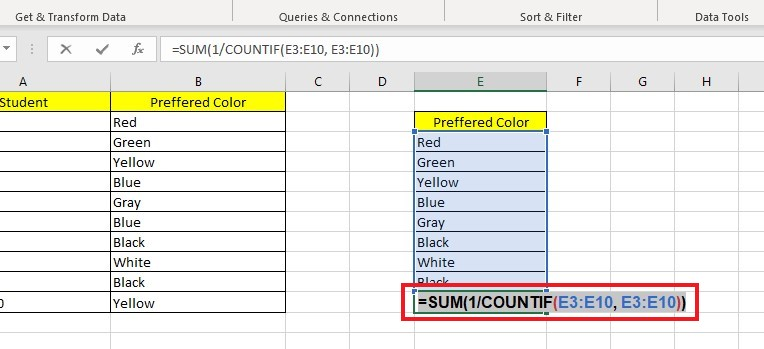1. Press Enter to get the result.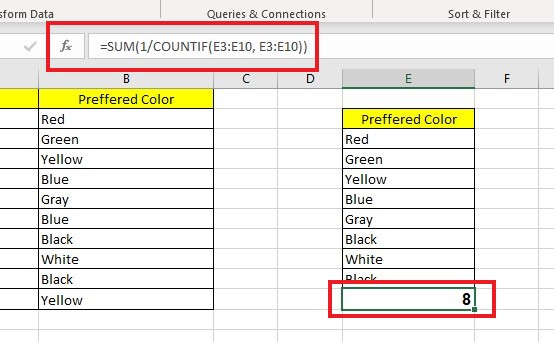Note: You can also tweak the formulae to find distinct text or numeric values.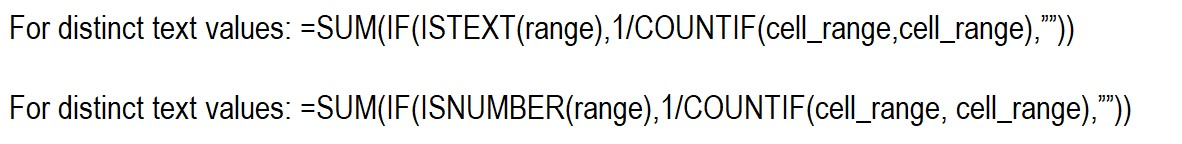## Final Thoughts on How to Count Unique Values in Excel

Counting unique values in Excel can be a great way to make sure your data is organized and consistent as you work with it. Knowing how to use the formulas available can make analyzing complex datasets easier, saving time and eliminating potential errors.

Visit Simple Sheets for more easy-to-follow guides, and remember to visit the related articles section of this blog post. For the most straightforward Excel video tutorials, subscribe to Simple Sheets on Youtube!## Frequently Asked Questions on How to Count Unique Values in Excel

### Why should I use the MATCH function when finding the unique values?

The MATCH function provides the position number of the first occurrence of each item in a data range. In case of duplicate values, the MATCH function will only return the position of the first occurrence in the data range.

### When should I use the FREQUENCY function when counting the unique values?

You can use the FREQUENCY function with numerical values. While it does count unique values, you can't use it with non-numeric data.

### Does a Pivot table help me to count unique values?

The Data Model, which integrates Power Pivot Table with Excel, offers users powerful features. One of these includes the ability for Pivot Tables to calculate the distinct counts of unique values in Excel.## Related Articles:

Learn How to Use the Excel Random Number Generator

Quick And Easy Guide On How To Total A Column in Excel

How To Add In Excel in 4 Easy Ways

#### Want to Make Excel Work for You? Try out 5 Amazing Excel Templates & 5 Unique Lessons

We hate SPAM. We will never sell your information, for any reason.